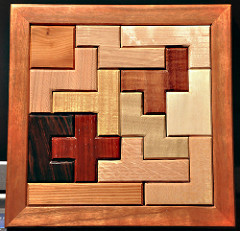# Polyomino Packing

Given a collection of polyominoes, pack them into a target shape.## Description

Given a collection of (not necessarily distinct) polyominoes and a target shape $S$, which is also a polyomino, the goal is to find a packing of the polyominoes into $S$, i.e., to place each polyomino inside the target shape so that no two polyominoes intersect. Notice that the area of $S$ might be larger than the sum of the areas of the polyominoes.

Depending on the version of the problem, horizontal or vertical reflection and rotations multiples of 90 degrees of the polyominoes might be allowed.

The picture shows $1$ tetromino and $12$ pentominoes packed in a $8 \times 8$ square.

## Computational complexity

• NP-complete even when the target shape is a square and the polyominoes are bounded by a rectangle of size $\Theta(\log N) \times \Theta(\log N)$  or $3 \times \Theta(\log N)$ , where $N$ is the total area of the polyominoes.

• Cannot be solved in time $2^{o(n / \log n)}$ unless the Exponential Time Hypothesis fails , even when:
• the polyominoes are bounded by $2 \times \Theta(\log n)$ rectangles and $S$ is a $3 \times n$ rectangle; or
• the polyominoes are bounded by $2 \times \Theta(\log n)$ rectangles and $S$ is bounded by a $2 \times n$ rectangle.
• Solvable in time $2^{O(N^{3/4} / \log N)}$ time, if $S$ is a $2 \times N$ rectangle .

• Solvable in time $2^{O(N / \log N)}$ time, if $S$ has area $N$ .

• Solvable in time $2^{O(\sqrt{N} \log N)}$ if the polyominoes are rectangular and $S$ is a rectangle of area $N$ .

The above results also hold when rotations and/or reflections are allowed.

 E. D. Demaine, M. L. Demaine. Jigsaw Puzzles, “Edge Matching, and Polyomino Packing: Connections and Complexity”, Graphs and Combinatorics, 2007.

 M. Brand, “Small polyomino packing”, Information Processing Letters, 2017.

 H. L. Bodlaender, T. C. van der Zanden, “On the Exact Complexity of Polyomino Packing”, in FUN 2018

Picture by Jeffrey Bary (CC BY 2.0).

@misc{cog:polyomino-packing,
author = "{CoG contributors}",
title  = "{Polyomino Packing --- Complexity of Games}",
year   = "2022",
url    = "https://www.isnphard.com/i/polyomino-packing/",
note   = "[Online; accessed 2022-06-02]"
}